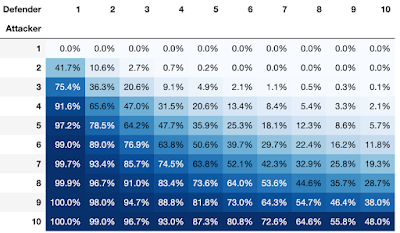## Thursday, September 17, 2020

### Game of Risk

The last assignment in the CIS194 Haskell class is to simulate dice-throwing battles in the game of Risk. The combat rules, taken from assignment specs, are:

• There is an attacking army (containing some number of units) and a defending army (containing some number of units).
• The attacking player may attack with up to three units at a time. However, they must always leave at least one unit behind. That is, if they only have three total units in their army they may only attack with two, and so on.
• The defending player may defend with up to two units (or only one if that is all they have).
• To determine the outcome of a single battle, the attacking and defending players each roll one six-sided die for every unit they have attacking or defending. So the attacking player rolls one, two, or three dice, and the defending player rolls one or two dice.
• The attacking player sorts their dice rolls in descending order. The defending player does the same.
• The dice are then matched up in pairs, starting with the highest roll of each player, then the second-highest.
• For each pair, if the attacking player’s roll is higher, then one of the defending player’s units die. If there is a tie, or the defending player’s roll is higher, then one of the attacking player’s units die.
We assume it is optimal to always attack or defend with as many units as possible.

Given an initial number of attacking and defending units, what is the expected outcome (say, the probability that the attacker wins)?

It's not obvious that there is a closed-form expression, but we can find programmatically the exact probabilities.

First, from the game specs, we know that there are only a few primitive battle patterns (A, D), where A is attacked and D is defender: (1, 1), (2, 1), (3, 1), (1, 2), (2, 2), (3, 2). For each pattern, we can generate all possible permutations of die values. Each permutation represent a battle, with an outcome ("loss scenario") in which A and D both lose some number of units (which can be zero units, if they win all dice rolls).

For example, for (A,D) of (1,1), both the attacker and defender role one die each, yielding 36 pairs of possible die rolls: (1,1), (1,2), (1,3) ... (6,5), (6,6). Tallying up the proportion of outcomes: A loses 1 unit 21 / 36; D loses 1 unit 15 / 36.

(Incidentally, the closed-form expression for 1 vs 1 is relatively simple: for each N that the defender rolls, with 1/6 probability each, the attacker loses in N/6 scenarios. For example, if the defender rolls 4, the attacker loses for all die values <= 4. So we can evaluate the expression (1/6) * (sum [1..6]) / 6, which equals 21/36. See these notes for a more detailed explanation.)

Once we have the loss scenarios with proportions for each primitive battle pattern, finding the exact win probability for the attacker is recursive: for any given starting pattern (A, D), lookup the relevant primitive battle pattern. For each loss scenario in which the attacker does not lose the game, update the battlefield with the losses and recursively lookup the next loss scenario, chaining the proportions (probabilities) until the game ends for each branch.

The win probabilities for the attacker for patterns up to (10, 10) are:Using Rational numbers in Haskell allows the answers to be expressed exactly. For example for (4,2), the win probability for A is 6610505 % 10077696 (see the rightmost column here).